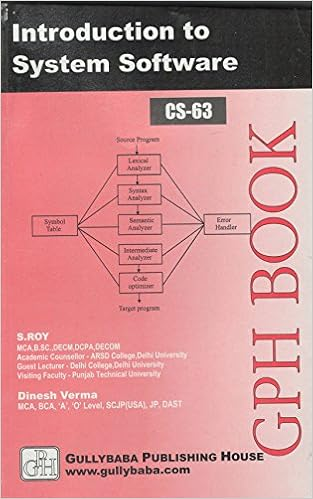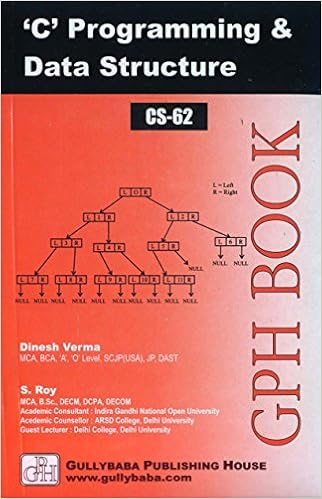IGNOU BCA MCA.comQuestion Papers of IGNOU BCA CS62

 CS62: 'C' programming and Data StructureYear: 2002 TEE: December Time 2 Full Marks 60 Note: There are 6 questions in this paper. Question 1 is compulsory. Answer any three questions from the rest. All algorithms should be written nearer to ?c? language.Q.1(a): Ackermann's function A(m,n) is defined as follows -Write a recursive algorithm for computing this function. Q.1(b): Let 'P' be a pointer to a doubly linked list. Show how this list may be used as a queue by writing algorithms to add and delete elements. Specify the value for 'P' when the queue is empty.: Q.1(c): Write a non-recursive procedure for traversing a Binary Tree in Postorder.: Q.2(a): Draw the internal memory representation of the following Binery Tree Using sequential representation:: home || Next >>